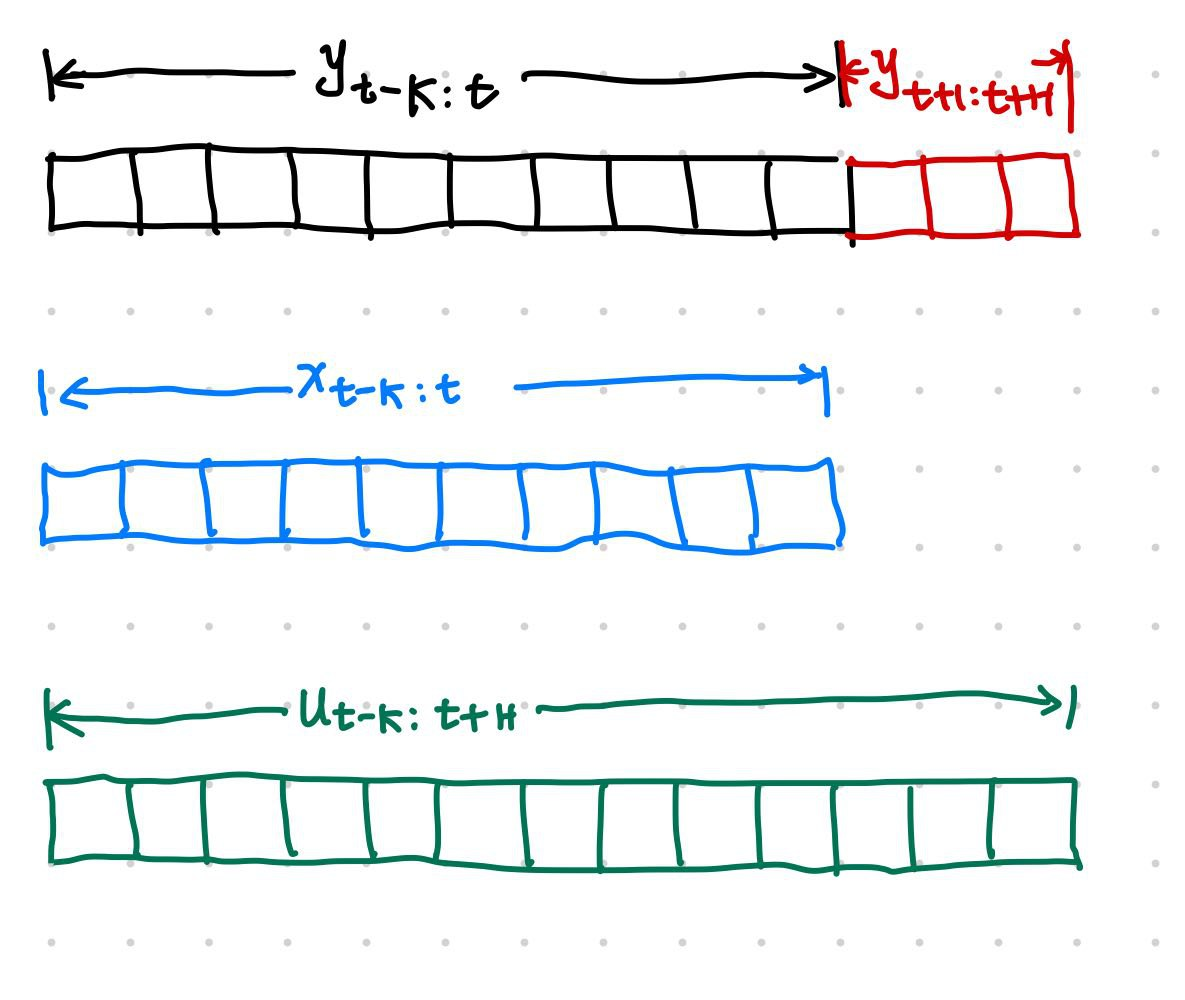# Time Series Data and Statistical Forecasting Mothods¶

## Time Series Data¶

Time series data comes from a variety of data generating processes. There are also different formulations and views of time series data.

Time series data can be formulated as a sequence of vector functions of time 1. There are many different types of tasks on time series data, for example,

• classification,
• anomaly detection, and
• forecasting.

In this chapter, we focus on the forecasting problem.

## The Forecasting Problem¶

To make it easier to formulate the forecasting problem, we group the time series features based on the role they play in a forecasting problem. Given a dataset $$\mathcal D$$, with

1. $$y^{(i)}_t$$, the sequential variable to be forecasted,
2. $$x^{(i)}_t$$, exogenous data for the time series data,
3. $$u^{(i)}_t$$, some features that can be obtained or planned in advance,

where $${}^{(i)}$$ indicates the $$i$$th variable, $${}_ t$$ denotes time. In a forecasting task, we use $$y^{(i)} _ {t-K:t}$$, $$x^{(i) _ {t-K:t}}$$, and $$u^{(i)} _ {t-K:t+H}$$, to forecast the future $$y^{(i)} _ {t+1:t+H}$$. In these notations, $$K$$ is the input sequence length and $$H$$ is the forecast horizon.A forecasting model $$f$$ will use $$x^{(i)} _ {t-K:t}$$ and $$u^{(i)} _ {t-K:t+H}$$ to forecast $$y^{(i)} _ {t+1:t+H}$$.

In the section Time Series Forecasting Tasks, we will discuss more details of the forecasting problem.

## Methods of Forecasting Methods¶

Januschowsk et al proposed a framework to classify the different forecasting methods2. We illustrate the different methods in the following charts.

flowchart TB

subgraph Objective

params_shared["Parameter Shared Accross Series"]

params_shared --"True"-->Global
params_shared --"False"-->Local

uncertainty["Uncertainty in Forecasts"]
uncertainty --"True"--> Probabilistic["Probabilistic Forecasts:\n forecasts with predictive uncertainty"]
uncertainty --"False"--> Point["Point Forecasts"]

computational_complexity["Computational Complexity"]

end

subgraph Subjective

structural_assumptions["Strong Structural Assumption"] --"Yes"--> model_driven["Model-Driven"]
structural_assumptions --"No"--> data_driven["Data-Driven"]

model_comb["Model Combinations"]

discriminative_generative["Discriminative or Generative"]

theoretical_guarantees["Theoretical Guarantees"]

predictability_interpretability["Predictability and Interpretibility"]

end

1. Dorffner G. Neural networks for time series processing. Neural Network World 1996; 6: 447–468.

2. Januschowski T, Gasthaus J, Wang Y, Salinas D, Flunkert V, Bohlke-Schneider M et al. Criteria for classifying forecasting methods. International journal of forecasting 2020; 36: 167–177.

Contributors: LM